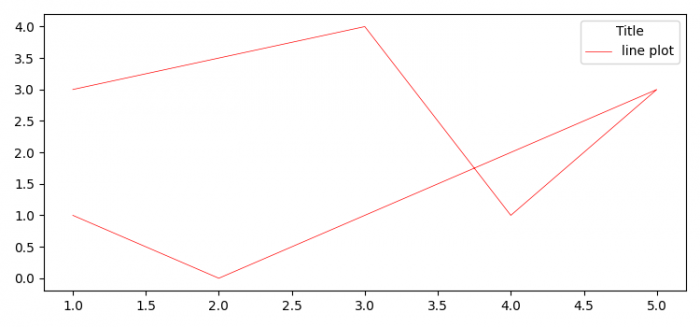# How to modify a Matplotlib legend after it has been created?

To modify a Matplotlib legend after it has been created, we can have multiple methods to modify the created legend.

• Set the figure size and adjust the padding between and around the subplots.
• Plot a line using plot() method, with two lists and a label.
• Use legend() method to place a legend over the plot.
• To modify the matplotlib legend, use set_title() method.
• To display the figure, use show() method.

## Example

from matplotlib import pyplot as plt

plt.rcParams["figure.figsize"] = [7.50, 3.50]
plt.rcParams["figure.autolayout"] = True

plt.plot([1, 3, 4, 5, 2, 1], [3, 4, 1, 3, 0, 1],
label="line plot", color='red', lw=0.5)

leg = plt.legend(loc="upper right")
leg.set_title("Title")

plt.show()

## Output